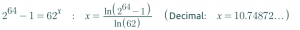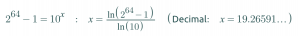# 短网址生成PHP函数base_convert可以在2和36进制之间转换，对于62进制就不行，这里分享一个Base62 的转换类，可以将10进制数字转换位62进制

```<?php

declare(strict_types=1);

namespace Dig\Conversion;

class Base
{
const BASE_MIN = 2;
const BASE_MAX = 62;

public function __construct(int \$base, string \$aplphabet)
{
if ((\$base < self::BASE_MIN) || (\$base > self::BASE_MAX)) {
throw new \Exception('base convert only require '.self::BASE_MIN.' <= base <= '.self::BASE_MAX);
}
if (empty(\$aplphabet)) {
throw new \Exception('cannot have empty aplphabet');
}
if (\$base > \strlen(\$aplphabet)) {
throw new \Exception('base convert only require base <= aplphabet length ');
}
\$this->base = \$base;
\$this->alphabet = \$aplphabet;
}

public function encode(int \$number): string
{
\$number = (string) \$number;
\$base = (string) \$this->base;
\$reminder = \bcmod(\$number, \$base);
\$quotient = \bcdiv(\$number, \$base);
\$result = \$this->alphabet[\$reminder];

while (\$quotient) {
\$reminder = \bcmod(\$quotient, \$base);
\$quotient = \bcdiv(\$quotient, \$base);
\$result = \$this->alphabet[\$reminder] . \$result;
}
return \$result;
}
public function decode(string \$number): int
{
\$base = (string) \$this->base;
\$length = \strlen(\$number);
\$result = (string) \strpos(\$this->alphabet, \$number);

for (\$i = 1; \$i < \$length; \$i++) {
\$result = \bcadd(\bcmul(\$base, \$result), (string) \strpos(\$this->alphabet, \$number[\$i]));
}
return (int)\$result;
}
}

class Base62 extends Base
{
public function __construct()
{
parent::__construct(62, '0123456789abcdefghijklmnopqrstuvwxyzABCDEFGHIJKLMNOPQRSTUVWXYZ');
}
}

```

```include __DIR__.'/../vendor/autoload.php';

use Dig\Conversion\Base62;

\$base62 = new Base62();
\$id = 56800235584;

for (\$i = 1; \$i < 100; \$i++) {
\$encode = \$base62->encode(\$id);
\$decode = \$base62->decode(\$encode);
echo \$id.'->'.\$encode.'->'.\$decode.PHP_EOL;
\$id += \$i;
}
```